# Kaleidoscopes

For the third time on cp4space, I’m going to refer to my Wythoffian polyhedron generator. This constructs a polyhedron by the use of some two-dimensional fundamental region, bounded by mirrors meeting at predetermined angles. For example, a triangle with internal angles of π/2, π/3 and π/5 generates polyhedra with icosahedral symmetry: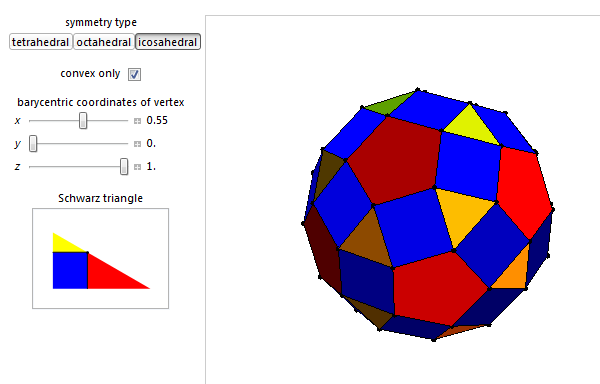Now, we can compute the Euler characteristic of a fundamental region, and use this to determine the number of copies of the fundamental region necessary to tile a surface of a particular genus. For instance, when the fundamental region is a triangle with internal angles of π/p, π/q and π/r, the Euler characteristic of the region is:$\chi = V + F - E = \dfrac{1}{2p} + \dfrac{1}{2q} + \dfrac{1}{2r} + 1 - \dfrac{3}{2}$

More generally, for an arbitrary polygon, we have:

• V is the sum of interior angles divided by 2π;
• E is half of the number of edges;
• F = 1.

In the icosahedral case, we have$\chi = \dfrac{1}{60}$, so we need 120 copies of the fundamental region to tile this sphere (which has an Euler characteristic of 2). Instantly, we can determine from a polygonal kaleidoscope whether it generates an elliptic, flat or hyperbolic tessellation, and determine how many times it covers the region.Let’s try another one, namely the hyperbolic triangle with interior angles of π/2, π/3 and π/7. This gives an Euler characteristic of$\chi = -\dfrac{1}{84}$. It can tile a three-holed torus (Euler characteristic −4) with 336 copies, arranged in a nice structure with automorphism group PGL(2,7).

What about using squares to tile a torus? This ends up being indeterminate, since the Euler characteristic of the torus and the square are both 0. The same applies to tiling a Klein bottle with hexagons, or a Euclidean plane with triangles.

### More complicated kaleidoscopes

To describe groups generated by reflections in higher dimensions, one generally uses a Coxeter-Dynkin diagram. I’ve briefly discussed these before, so I’ll not reintroduce these remarkable objects. Instead, we shall concentrate on simply-laced Dynkin diagrams (all edges are unlabelled) that are simply-connected and have at most one vertex with degree greater than two. For example, the E8 diagram has branches of length 2, 3 and 5: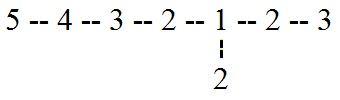These are analogous to the 2, 3 and 5 in the dodecahedral symmetry group, and the analogy is related to the McKay correspondence. It’s also mentioned here, although we’ll approach it with slightly more generality. We associate a Dynkin diagram with one multivalent vertex with a polygon, whose internal angles are given by π/l, where l is the length of the branch. Hence, the D4 affine diagram corresponds to a rectangular fundamental region: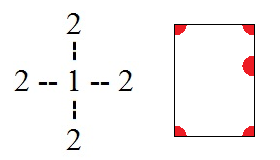It’s also well defined, since measuring angles of π on the edges of the polygon corresponds to branches of length 1 (the central node in the diagram). The thing that really makes this connection natural is the following:

• The Dynkin diagram is finite/affine/hyperbolic precisely when the surface generated by the two-dimensional kaleidoscope is spherical/flat/hyperbolic, respectively.

So, the rectangular kaleidoscope *2222 (in Thurston’s orbifold notation) corresponds to the D4 lattice, and they both generate flat tilings. Other correspondences are below:

Spherical:

• Dihedral symmetry *22n ↔ D(n+2)
• Tetrahedral symmetry *332 ↔ E6
• Octahedral symmetry *432 ↔ E7
• Icosahedral symmetry *532 ↔ E8

Flat:

• *2222 ↔ affine D4
• *333 ↔ affine E6
• Square tiling symmetry *442 ↔ affine E7
• Hexagonal honeycomb symmetry *632 ↔ affine E8

Hyperbolic:

• *732 ↔ hyperbolic E9 lattice
• *666 ↔ hyperbolic Coxeter group containing the bimonster as a quotient

Unfortunately, I can’t see how this would generalise to the affine Dn diagram (for n >= 5), since the diagram has two vertices of degree 3. Similarly, this doesn’t work particularly well for the regular An diagram, which is just a straight line:

• A7 = 4—3—2—1—2—3—4

So, according to our correspondence, this gives this hosohedron: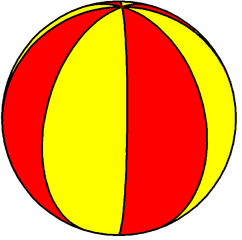Now, there is a certain ambiguity in the construction. For example, what if we had labelled A7 using an off-centre root node?

• A7 = 5—4—3—2—1—2—3

This would correspond to a digonal fundamental region with angles of π/3 and π/5, which just doesn’t work properly. Hence, we had no choice but to choose the centre node. A more awkward repercussion is that this is only possible for odd n. For example, the hosohedra for A3 and A5 are shown below, but the construction fails for A4.Well, that is at least suggestive of what A4 should correspond to: a hosohedron with five lunes, alternately coloured. When one attempts to construct it, serious difficulties are encountered. However, we can avoid those complications by using the double cover of the spherical symmetry group, in which case we recover the original McKay correspondence.

We can also make sense of some infinite Coxeter diagrams. For example, the E∞ diagram (limit of En for all n, which gives an infinitely-generated Coxeter group) below corresponds to the *23∞ symmetry group (isomorphic to the modular group PGL(2,Z)):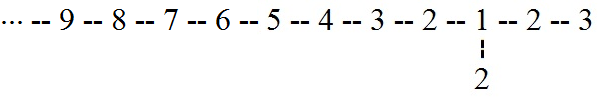I don’t think that this analogy between Dynkin diagrams and two-dimensional kaleidoscopes is anything other than a convenient mathematical coincidence, although it seems to be intimately related to the McKay correspondence.

This entry was posted in Uncategorized. Bookmark the permalink.

### 0 Responses to Kaleidoscopes

1.juanmarqz says:

supercool info (y)

2.k says:

shameless self promotion 😀 :
3.matthew says: